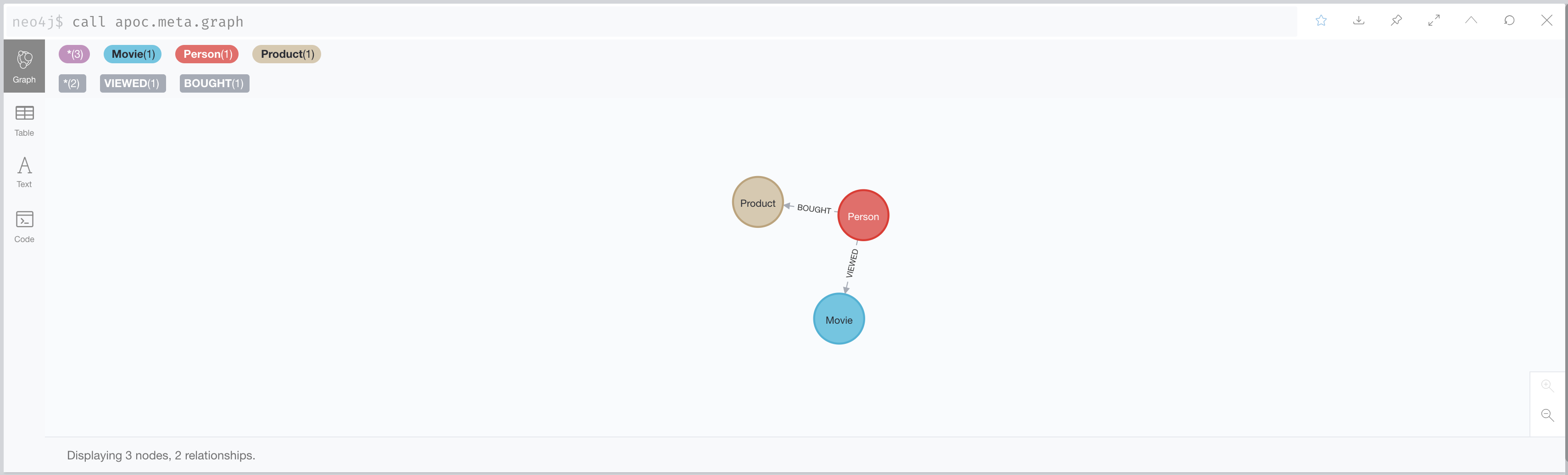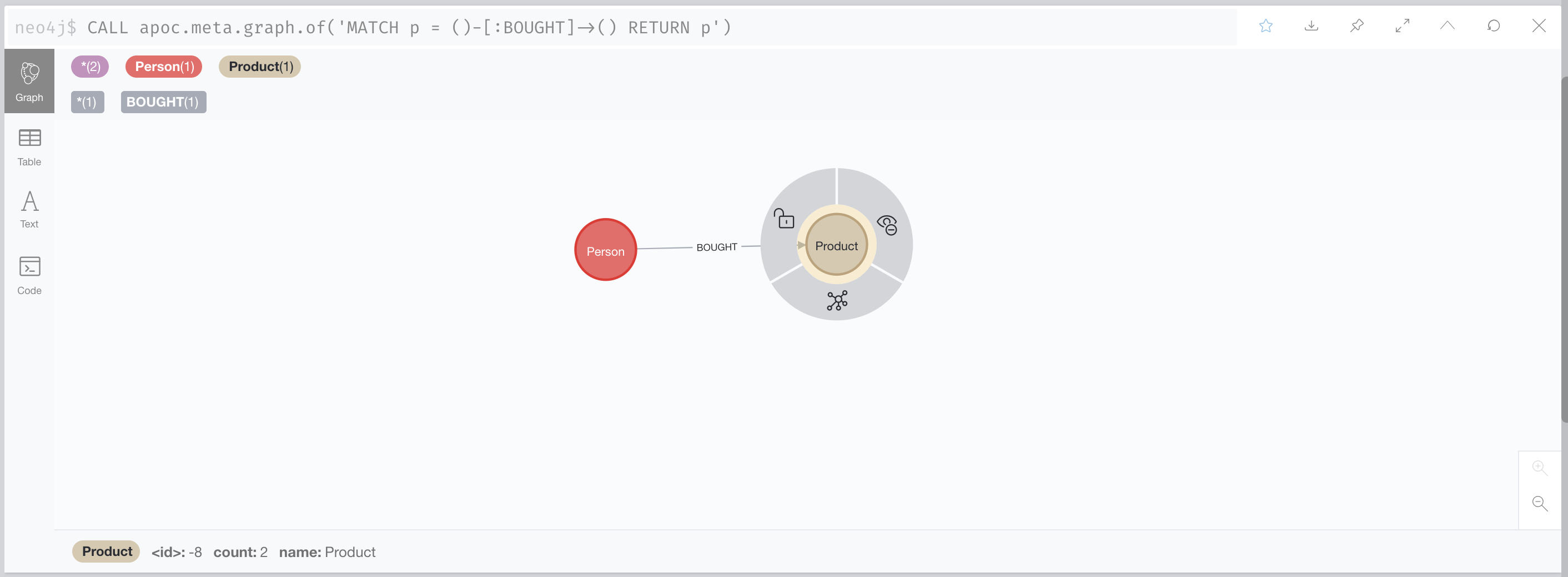# apoc.meta.graph.of

Procedure APOC Core

apoc.meta.graph.of({graph}, {config}) - examines a subset of the graph to provide a graph meta information

## Signature

``apoc.meta.graph.of(graph = {} :: ANY?, config = {} :: MAP?) :: (nodes :: LIST? OF NODE?, relationships :: LIST? OF RELATIONSHIP?)``

## Input parameters

Name Type Default

graph

ANY?

{}

config

MAP?

{}

## Config parameters

This procedure supports the following config parameters:

Table 1. Config parameters
Name Type Default Description

sample

Long

1

Number of nodes to skip, e.g. a sample of 1000 will read every 1000th node. Defaults to read every node.

maxRels

Long

-1

Number of relationships to read per sampled node. A value of -1 will read all.

## Output parameters

Name Type

nodes

LIST? OF NODE?

relationships

LIST? OF RELATIONSHIP?

## Sampling

This procedure works by using the database statistics. A new node is returned for each label, and its connecting relationships are calculated based on the pairing combinations of [:R]→(:N) and (:M)→[:R]. For example, for the graph (:A)-[:R]→(:B)-[:R]→(:C), the path (:B)-[:R]→(:B) will be calculated from the combination of [:R]→(:B) and (:B)-[:R]. This procedure will post-process the data by default, removing all non-existing relationships. This is done by scanning the nodes and their relationships. If the relationship is not found, it is removed from the final result. This slows down the procedure, but will produce an accurate schema.

See apoc.meta.graphSample to avoid performing any post-processing.

It is also possible to specify how many nodes and relationships to scan. The config parameter `sample` gives the skip count, and the `maxRels` parameter gives the max number of relationships that will be checked per node. If `sample` is set to 100, this means that every 100th node will be checked per label, and a value of 100 for `maxRels` means that for each node read, only the first 100 relationships will be read. Note that if these values are set, and the relationship is not found within those constraints, it is assumed that the relationship does not exist, and this may result in false negatives.

A `sample` value higher than the number of nodes for that label will result in one node being checked.

## Type of supported input graphs

Type Description

String

a Cypher query

Virtual Graph

a Virtual Graph returned by `apoc.graph.*`

Map

a map with two field `nodes` (a list of nodes, mandatory), `relationships` (a list of relationships)

If you have a quite complex Graph, and you want to analyze and get some info about a specific sub-graph in it, you can leverage the `apoc.meta.graph.of` procedure.

So for the given Graph Model:You can leverage the `apoc.meta.graph.of` procedure in this way:That will extract the Meta Graph of the provided query, with some stats like the `count` for each node involved into the query.

If you want more details you can also look at `apoc.meta.graph` documentation